## Tau Function

A functionrelated to the Divisor Function, also sometimes called Ramanujan's Tau Function. It is given by the Generating Function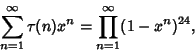(1)

and the first few values are 1,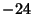, 252,, 4380, ... (Sloane's A000594).is also given by(2)(3)(4)

In Ore's Conjecture, the tau function appears as the number of Divisors of. Ramanujanconjectured and Mordell proved that if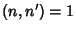, then(5)

Ramanujanconjectured and Watson proved thatis divisible by 691 for almost all. If(6)

then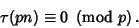(7)

Values offor which the first equation holds are, 3, 5, 7, 23.

Ramanujanalso studied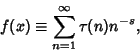(8)

which has properties analogous to the Riemann Zeta Function. It satisfies(9)

and Ramanujan'sTau-Dirichlet Series conjecture alleges that all nontrivial zeros oflie on the line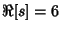.can be split up into(10)

where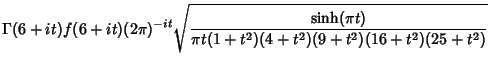(11)(12)

The Summatory tau function is given by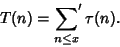(13)

Here, the prime indicates that whenis an Integer, the last termshould be replaced by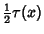.

Ramanujan's tau theta function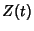is a Real function for Realand is analogous to the Riemann-Siegel Function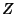. The number of zeros in the critical strip from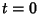tois given by(14)

whereis the Riemann Theta Function andis the Tau-Dirichlet Series, defined by(15)

Ramanujanconjectured that the nontrivial zeros of the function are all real.

Ramanujan'sfunction is defined by(16)

whereis the Tau-Dirichlet Series.

Hardy, G. H. Ramanujan's Function.'' Ch. 10 in Ramanujan: Twelve Lectures on Subjects Suggested by His Life and Work, 3rd ed. New York: Chelsea, 1959.
Sloane, N. J. A. Sequence A000594/M5153 in An On-Line Version of the Encyclopedia of Integer Sequences.'' http://www.research.att.com/~njas/sequences/eisonline.html and Sloane, N. J. A. and Plouffe, S. The Encyclopedia of Integer Sequences. San Diego: Academic Press, 1995.